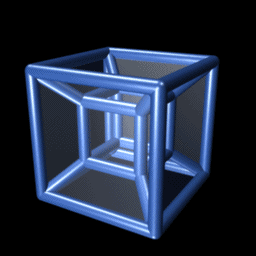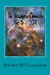## The quantum properties of non-point particles

We know that everything in the universe including particles have physical size.

Even so for the past 50 years, the Standard Model of particle physics which many say has given us the most complete mathematical description of the particles and forces that shape our world ignores this fact and treats them all as size less dimensionless mathematical points.

Many physicists feel this way because it predicts with so much accuracy the microscopic properties of particles and the macroscopic ones of stars and galaxies that it must be a correct physical model that even though as was just mentioned it treats all particles and their interactions not in term of their physical size but in terms of mathematic points.

However in 1924 Louis de Broglie’s showed that it cannot be when he theorized that all particle’s have a wave component and that one must take this into account when one defines how they interact with their environments.  This fact becomes irrefutable when in 1927 Davisson and Germer observed that electrons were diffracted by crystals.  Later it was determined the equation E = hν which defines the wavelength and therefore the physical volume occupied by a particle could be used to calculate the magnitude of that diffraction. In other words one must take into consideration the physical size of a particle to determine how they interact with a crystal.

This means that one cannot assume as the Standard Model does that a particle can be defined as points and expect to develop a complete description of how and why force and particles interact in our observable environment.

In other words one to understand the properties of point particles one must take into consideration their spatial extended properties.

For example in the article "Why is energy/mass quantized?" Oct. 4, 2007 where it was shown the quantum mechanical properties of particles can be defined by extrapolating the laws classical resonance in a three-dimensional environment to a matter wave on a "surface" of a three-dimensional space manifold with respect to a fourth *spatial* dimension.

(Einstein gave us the ability to do this when he defined geometric properties of a space time universe in terms of the equation E=mc^2 and the constant velocity of light. This is because it allows one to redefine a unit of time he associated with energy in his space-time universe to unit of space in one consisting of only four *spatial* dimensions. )

Briefly it showed the four conditions required for resonance to occur in a classical environment, an object, or substance with a natural frequency, a forcing function at the same frequency as the natural frequency, the lack of a damping frequency and the ability for the substance to oscillate spatial would be meet by a matter wave in four spatial dimensions.

The existence of four *spatial* dimensions would give a matter wave the ability to oscillate spatially on a "surface" between a third and fourth *spatial* dimensions thereby fulfilling one of the requirements for classical resonance to occur.

These oscillations would be caused by an event such as the decay of a subatomic particle or the shifting of an electron in an atomic orbital.  This would force the "surface" of a three-dimensional space manifold with respect to a fourth *spatial* dimension to oscillate with the frequency associated with the energy of that event.

The oscillations caused by such an event would serve as forcing function allowing a resonant system or "structure" to be established in four *spatial* dimensions.

Classical mechanics tells us the energy of a resonant system can only take on the discrete or quantized values associated with its resonant or a harmonic of its resonant frequencyTherefore the discrete or quantized energy of resonant systems is responsible for the discrete quantized quantum mechanical properties of particles.

However, it did not explain how the boundaries of a particle’s resonant structure are created in free space.

In other words why is electromagnetic energy not perceived to have the properties of a continues wave moving though space but those of particle

In classical physics, a point on the two-dimensional surface of paper is confined to that surface.  However, that surface can oscillate up or down with respect to three-dimensional space.

Similarly an object occupying a volume of three-dimensional space would be confined to it however, it could, similar to the surface of the paper oscillate "up" or "down" with respect to a fourth *spatial* dimension.

The confinement of the "upward" and "downward" oscillations of a three-dimension volume with respect to a fourth *spatial* dimension is what defines the geometric boundaries of the resonant system associated with a particle in the article "Why is energy/mass quantized?"

However one can also understand why we perceive there locations in terms of the probabilities associated with quantum mechanics.

The reason why we do not observe energy in its extended wave form is that, as mentioned earlier all energy is propagated through space in discrete components associated with its resonant structure.  Therefore, its energy appears to originate from a specific point in space associated with where an observer samples or observes that that energy.

This is analogous to how the energy of water in a sink is release by allowing it to go down the drain.  If all we could observe is the water coming out of the drain we would have to assume that it was concentrated in the region of space defined by the diameter of the drain.  However, in reality the water occupies a much larger region.

However this also gives one the ability to understand in terms of a physical image the probabilistic interpretation of quantum mechanic interns of where the energy of this matter wave is obverse or measured.

Classical wave mechanics tells us a wave’s energy is instantaneously constant at its peaks and valleys or the 90 and 270-degree points as its slope changes from positive to negative while it changes most rapidly at the 180 and 360-degree points.

Therefore, the precise position of a particle could be only be defined at the "peaks" and "valleys" of the matter wave responsible for its resonant structure because those points are the only place where its energy or "position" is stationary with respect to a fourth *spatial* dimension.  Whereas its precise momentum would only be definable with respect to where the energy change or velocity is maximum at the 180 and 360-degree points of that wave.  All points in between would only be definable in terms of a combination of its momentum and position.

However, to measure the exact position of a particle one would have to divert or "drain" all of the energy at the 90 or 270-degree points to the observing instrument leaving no energy associated with its momentum left to be observed by another instrument.  Therefore, if one was able to precisely determine position of a particle he could not determine anything about its momentum.  Similarly, to measure its precise momentum one would have to divert all of the energy at the 180 or 360 point of the wave to the observing instrument leaving none of its position energy left to for an instrument which was attempting to measure its position.  Therefore, if one was able to determine a particles exact momentum one could not say anything about its position.

The reason we observe a particle as a point mass instead of an extended wave is because, as mentioned earlier the article when we observe or "drain" the energy continued in its wave function, whether it be related to its position or momentum it will appear to come from a specific point in space similar how the energy of water flowing down a sink drain appears to be coming from a "point" source with respect the extended volume of water in the sink.

As mentioned earlier, all points in-between are a dynamic combination of both position and momentum.  Therefore, the degree of accuracy one chooses to measure one will affect the other.

For example, if one wants to measure the position of a particle to within a certain predefined distance "m" its wave energy or momentum will have to pass through that opening.  However, Classical Wave Mechanics tells us that as we reduce the error in our measurement by decreasing the predefine distance interference will cause its energy or momentum to be smeared our over a wider area thereby making its momentum harder to determine.  Summarily, to measure its momentum "m"kg / s one must observe a portion the wavelength associated with its momentum.  However, Classical wave mechanics tell us we must observe a larger portion of its wavelength to increase the accuracy of the measurement of its energy or momentum.  But this means that the accuracy of its position will be reduced because the boundaries determining its position within the measurement field are greater.

However, this dynamic interaction between the position and momentum component of the matter wave would be responsible for the uncertainty Heisenberg associated with their measurement because it shows the measurement of one would affect the other by the product of those factors or m^2 kg / s.

Yet because of the time varying nature of a matter wave one could only define its specific position or momentum of a particle based on the amplitude or more precisely the square of the amplitude of its matter wave component.

This shows that one can develop a complete description for how particles can exist as a point as the Standard Model assumes they do while at the same time have the spatial properties need to define our reality

Later Jeff

 Anthology of The Imagineer’s Chronicles Vol. 1 thru 7 2007 thru 2016Ebook \$10.50 The Reality of the Fourth Spatial Dimension  Paperback \$9.77 Ebook    \$6.24 The Imagineer’s Chronicles Vol. 7 — 2016Paperback \$11.25 E-book \$7.25 The Imagineer’s Chronicles Vol. 6 — 2015Paperback \$12.25 E-book \$9.89Paperback \$14.84 Ebook \$9.97 The Imagineer’s Chronicles Vol. 2 — 2011Paperback \$9.24 Ebook \$6.57 The Imagineer’s Chronicles 2007 thru 2010Paperback \$15.43 Ebook \$7.82see more...